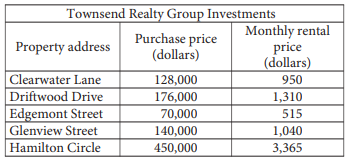mycollegehive
The Townsend Realty Group invested in the five different p...
math SAT SAT-math-test0The Townsend Realty Group invested in the five different properties listed in the table above. The table shows the amount, in dollars, the company paid for each property and the corresponding monthly rental price, in dollars, the company charges for the property at each of the five locations.

The relationship between the monthly rental price r, in dollars, and the property’s purchase price p, in thousands of dollars, can be represented by a linear function. Which of the following functions represents the relationship?

A) r(p) = 2.5p - 870

B) r(p) = 5p + 165

C) r(p) = 6.5p + 440

D) r(p) = 7.5p - 10

1.8K viewsShareFollowAsked by judziyrep:1.9K
University of Benin Nigeria
28 May 2020

Comments

### 2 answer(s)

Advertisement (continue below)Peter answeredrep:1.7K
University of Lagos Nigeria
14 August 20200Since the relationship is linear, the straight line equation, y = mc + c is valid.

The equation can be written in (p, r(p)) cordinates as:

r(p) = mp + c ---------- eqn(1)

Where m is the slope and c is the intercept

m = y2 - y1 / x2 - x1

picking any two points from the table above,

(128000, 950) (176000 , 1310)

The above points when the property’s purchase price is in thousands of dollars is given as: (128, 950) and (176 , 1310)

m = (1310 - 950) / (176 - 128)

m = 7.5

substitute m = 7.5 and cordinate (128, 950) into eqn(1)

950 = 7.5(128) + c

950 = 960 + c

subtract 960 from both sides

c = 950 - 960

c = -10.

Thus, the eqn(1) becomes

r(p) = 7.5p - 10Share
Comments

Advertisement (continue below)Peter answeredrep:1.7K
University of Lagos Nigeria
12 August 20200r(p) = 7.5p - 10

Ans DShare
Comments

### Groups

Advertisement (continue below)

Your Answer

How to insert math formulas/equations### Related Tags

math

20 followers

1261 questionsSAT

7 followers

487 questionsSAT-math-test

13 followers

476 questions